# 【华为云技术分享】【Python算法】分类与预测——Python随机森林

1.随机森林定义
随机森林是一种多功能的机器学习算法，能够执行回归和分类的任务。同时，它也是一种数据降维手段，在处理缺失值、异常值以及其他数据探索等方面，取得了不错的成效。另外，它还担任了集成学习中的重要方法，在将几个低效模型整合为一个高效模型时大显身手。在随机森林中，会生成很多的决策树，当在基于某些属性对一个新的对象进行分类判别时，随机森林中的每一棵树都会给出自己的分类选择，并由此进行“投票”，森林整体的输出结果将会是票数最多的分类选项；而在回归问题中，随机森林的输出将会是所有决策树输出的平均值。

2.随机森林的优缺点
该算法的优点主要有以下几个方面：
随机森林算法能解决分类与回归两种类型的问题，并在这两个方面都有相当好的估计表现；
随机森林对于高维数据集的处理能力令人兴奋，它可以处理成千上万的输入变量，并确定最重要的变量，因此被认为是一个不错的降维方法。此外，该模型能够输出变量的重要性程度，这是一个非常便利的功能；
在对缺失数据进行估计时，随机森林是一个十分有效的方法。就算存在大量的数据缺失，随机森林也能较好地保持精确性；
当存在分类不平衡的情况时，随机森林能够提供平衡数据集误差的有效方法；

模型的上述性能可以被扩展运用到未标记的数据集中，用于引导无监督聚类、数据透视和异常检测；
随机森林算法中包含了对输入数据的重复自抽样过程，即所谓的bootstrap抽样。

这样一来，数据集中大约三分之一将没有用于模型的训练而是用于测试，这样的数据被称为out of bag samples，通过这些样本估计的误差被称为out of bag error。研究表明，这种out of bag方法的与测试集规模同训练集一致的估计方法有着相同的精确程度，因此在随机森林中我们无需再对测试集进行另外的设置。

(1)随机森林在解决回归问题时并没有像它在分类中表现的那么好，这是因为它并不能给出一个连续型的输出。
(2)当进行回归时，随机森林不能够作出超越训练集数据范围的预测，这可能导致在对某些还有特定噪声的数据进行建模时出现过度拟合。
(3)对于许多统计建模者来说，随机森林给人的感觉像是一个黑盒子—几乎无法控制模型内部的运行，只能在不同的参数和随机种子之间进行尝试。

3.随机森林执行步骤

在随机森林中，每一个决策树“种植”和“生长”的规则如下所示：
(1) 假设训练集中的样本个数为N，然后通过有重置的重复多次抽样来获得这N个样 本，这样的抽样结果将作为生成决策树的训练集；
(2) 如果有M个输入变量，每个节点都将随机选择m(m<M)个特定的变量，然后运用这m个变量来确定最佳的分裂点。在决策树的生成过程中，m的值是保持不变的；
(3) 每棵决策树都最大可能地进行生长而不进行剪枝；
(4) 通过对所有的决策树进行加总来预测新的数据（在分类时采用多数投票，在回归时 采用平均）。

4.操作系统

操作机：Linux_Ubuntu
操作机默认用户：root

5.实验工具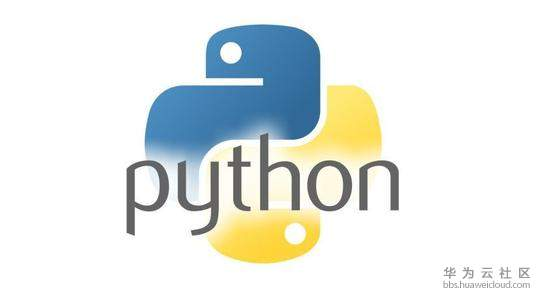Python是一种计算机程序设计语言。是一种动态的、面向对象的脚本语言，最初被设计用于编写自动化脚本(shell)，随着版本的不断更新和语言新功能的添加，越来越多被用于独立的、大型项目的开发。Python已经成为最受欢迎的程序设计语言之一。自从2004年以后，python的使用率呈线性增长。2011年1月，它被TIOBE编程语言排行榜评为2010年度语言。

众多开源的科学计算软件包都提供了Python的调用接口，例如著名的计算机视觉库OpenCV、三维可视化库VTK、医学图像处理库ITK。而Python专用的科学计算扩展库就更多了，例如如下3个十分经典的科学计算扩展库：NumPy、SciPy和matplotlib，它们分别为Python提供了快速数组处理、数值运算以及绘图功能。因此Python语言及其众多的扩展库所构成的开发环境十分适合工程技术、科研人员处理实验数据、制作图表，甚至开发科学计算应用程序。

6.Numpy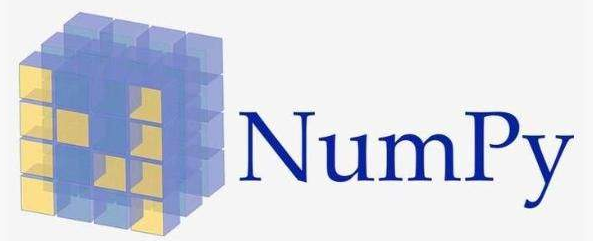NumPy系统是Python的一种开源的数值计算扩展。这种工具可用来存储和处理大型矩阵，比Python自身的嵌套列表（nested list structure)结构要高效的多（该结构也可以用来表示矩阵（matrix））。

NumPy（Numeric Python）提供了许多高级的数值编程工具，如：矩阵数据类型、矢量处理，以及精密的运算库。专为进行严格的数字处理而产生。多为很多大型金融公司使用，以及核心的科学计算组织如：Lawrence Livermore，NASA用其处理一些本来使用C++，Fortran或Matlab等所做的任务。

7.scikit-learnscikit-learn，Python 中的机器学习，简单高效的数据挖掘和数据分析工具，可供大家使用，可在各种环境中重复使用，建立在 NumPy，SciPy 和 matplotlib 上开放源码，可商业使用 - BSD license。

8.MatplotlibMatplotlib 是一个 Python 的 2D绘**，它以各种硬拷贝格式和跨平台的交互式环境生成出版质量级别的图形。通过 Matplotlib，开发者可以仅需要几行代码，便可以生成绘图，直方图，功率谱，条形图，错误图，散点图等。

9.pandas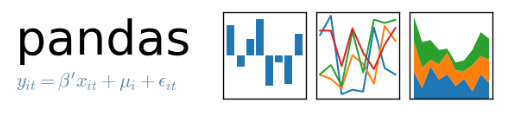Python Data Analysis Library 或 pandas 是基于NumPy 的一种工具，该工具是为了解决数据分析任务而创建的。Pandas 纳入了大量库和一些标准的数据模型，提供了高效地操作大型数据集所需的工具。pandas提供了大量能使我们快速便捷地处理数据的函数和方法。你很快就会发现，它是使Python成为强大而高效的数据分析环境的重要因素之一。

10.导入所需要的库:numpy,matplotlib.pyplot,pandas

# Importing the libraries
import numpy as np
import matplotlib.pyplot as plt
import pandas as pd

11.加载数据集

# Importing the dataset
X = dataset.iloc[:, [2, 3]].values
y = dataset.iloc[:, 4].values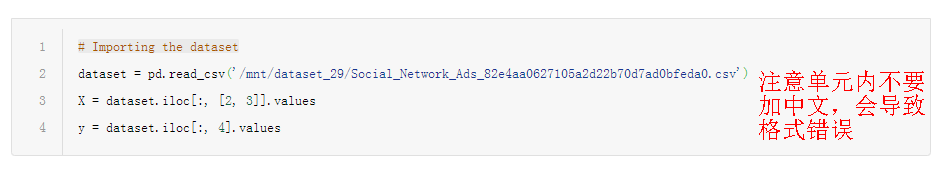12.将数据集分割为训练集和测试集

# Splitting the dataset into the Training set and Test set
from sklearn.model_selection import train_test_split
X_train, X_test, y_train, y_test = train_test_split(X, y, test_size = 0.25, random_state = 0)

13.特征缩放

# Feature Scaling
from sklearn.preprocessing import StandardScaler
sc = StandardScaler()
X_train = sc.fit_transform(X_train)
X_test = sc.transform(X_test)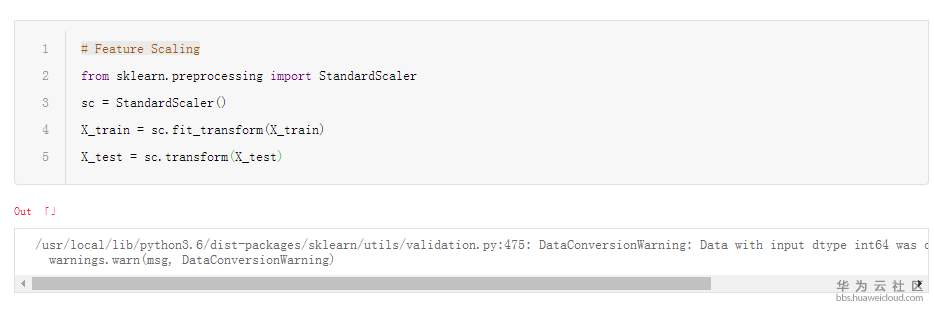14.调试训练集的随机森林

from sklearn.ensemble import RandomForestClassifier
classifier = RandomForestClassifier(n_estimators = 10, criterion = 'entropy', random_state = 0)
classifier.fit(X_train, y_train)

15.对测试集进行分类

# Predicting the Test set results
y_pred = classifier.predict(X_test)

16.制作混淆矩阵来评估模型性能

# Making the Confusion Matrix
from sklearn.metrics import confusion_matrix
cm = confusion_matrix(y_test, y_pred)

17.绘制训练数据分类结果

# Visualising the Training set results
from matplotlib.colors import ListedColormap
X_set, y_set = X_train, y_train
X1, X2 = np.meshgrid(np.arange(start = X_set[:, 0].min() - 1, stop = X_set[:, 0].max() + 1, step = 0.01),
np.arange(start = X_set[:, 1].min() - 1, stop = X_set[:, 1].max() + 1, step = 0.01))
plt.contourf(X1, X2, classifier.predict(np.array([X1.ravel(), X2.ravel()]).T).reshape(X1.shape),
alpha = 0.75, cmap = ListedColormap(('red', 'green')))
plt.xlim(X1.min(), X1.max())
plt.ylim(X2.min(), X2.max())
for i, j in enumerate(np.unique(y_set)):
plt.scatter(X_set[y_set == j, 0], X_set[y_set == j, 1],
c = ListedColormap(('orange', 'blue'))(i), label = j)
plt.title('Classifier (Training set)')
plt.xlabel('Age')
plt.ylabel('Estimated Salary')
plt.legend()
plt.show()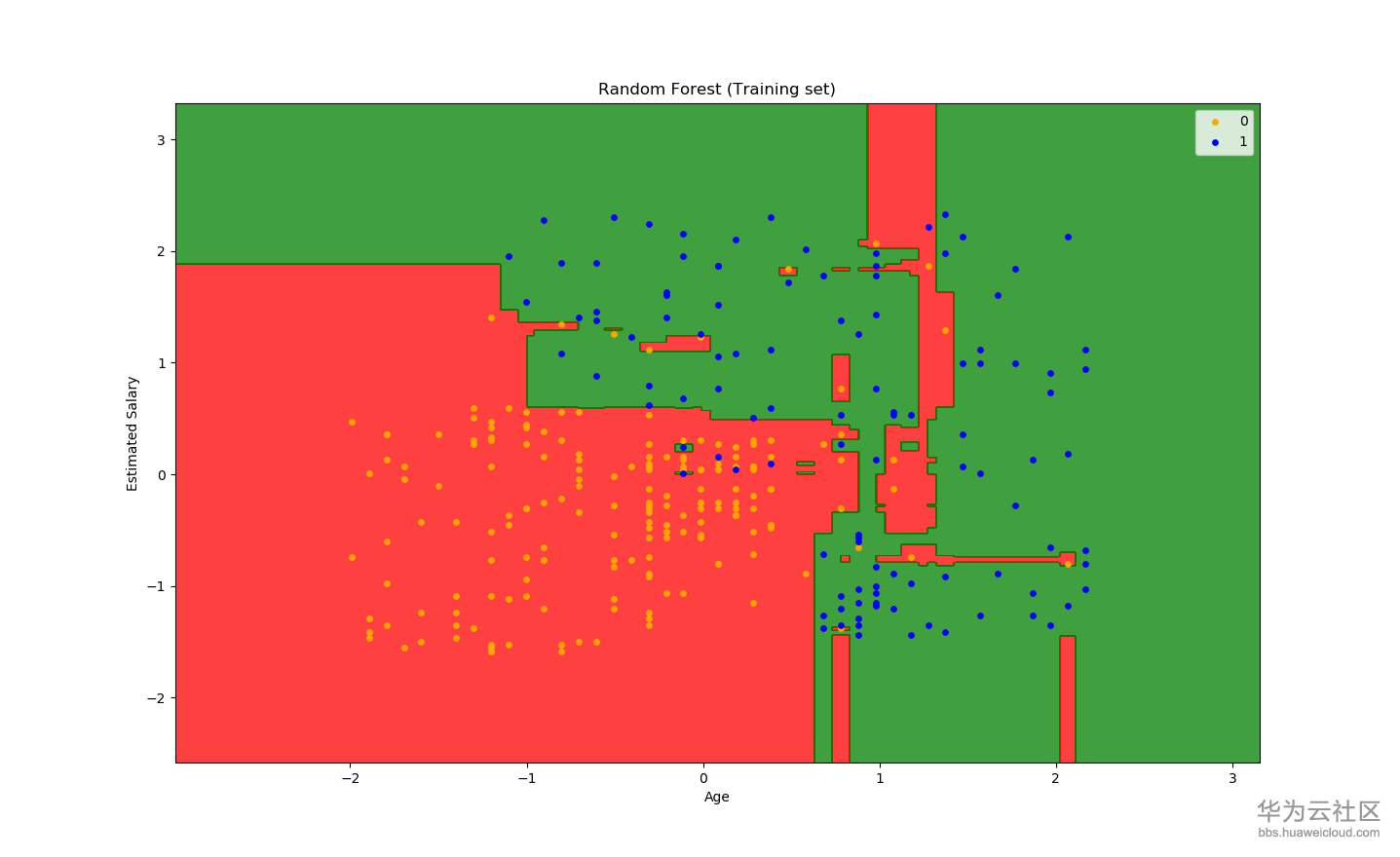18.绘制测试数据分类结果

# Visualising the Test set results
from matplotlib.colors import ListedColormap
X_set, y_set = X_test, y_test
X1, X2 = np.meshgrid(np.arange(start = X_set[:, 0].min() - 1, stop = X_set[:, 0].max() + 1, step = 0.01),
np.arange(start = X_set[:, 1].min() - 1, stop = X_set[:, 1].max() + 1, step = 0.01))
plt.contourf(X1, X2, classifier.predict(np.array([X1.ravel(), X2.ravel()]).T).reshape(X1.shape),
alpha = 0.75, cmap = ListedColormap(('red', 'green')))
plt.xlim(X1.min(), X1.max())
plt.ylim(X2.min(), X2.max())
for i, j in enumerate(np.unique(y_set)):
plt.scatter(X_set[y_set == j, 0], X_set[y_set == j, 1],
c = ListedColormap(('orange', 'blue'))(i), label = j)
plt.title('Classifier (Test set)')
plt.xlabel('Age')
plt.ylabel('Estimated Salary')
plt.legend()
plt.show()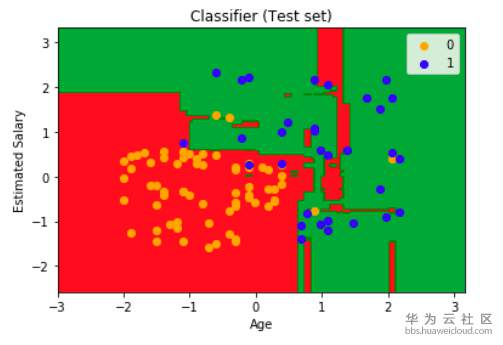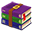社交网络测试数据集.csv.zip08-05980

#### 数据库实践丨MySQL多表join分析

05-068664

#### 【华为云技术分享】物联网常用开发板

01-211万+

#### 华为IoT首席架构师王启军：全栈工程师“养成记”

09-2314万+

#### 100 个网络基础知识普及，看完成半个网络高手

08-1033

#### 内存总是不够？HBase&GeoMesa配置优化了解一下

08-1037

#### 云图说 | 3分钟创建一个游戏类工作负载

08-1048

#### 【API进阶之路】帮公司省下20万调研费！如何巧用情感分析API实现用户偏好调研

08-1034

#### 多角度分析，通讯时序数据的预测与异常检测挑战

08-07236

#### 这些都是当年在C/C++上折的地儿…

08-07184点击重新获取扫码支付1.余额是钱包充值的虚拟货币，按照1:1的比例进行支付金额的抵扣。
2.余额无法直接购买下载，可以购买VIP、C币套餐、付费专栏及课程。余额充值